# Angle Cut Calculator

Created by Kenneth Alambra
Reviewed by Dominik Czernia, PhD and Jack Bowater
Last updated: Nov 20, 2022

This angle cut calculator, or woodworking angle finder, will help you determine the dimensions of angled planks used as knee bracing for your framing projects. In this calculator, you will learn what knee bracing is in structures and how to cut wood at an angle for bracing purposes.

Knowing how to calculate angle cuts and using basic algebra and trigonometry are great things to learn even if you already know how to measure angle cuts in wood using a protractor.

This calculator will help you start cutting angles for tightly fitting knee bracings in no time. Although we focus on knee bracing applications in woodworks, you can also use knee bracings in steel structures. Keep on reading to get started!

## What is a knee bracing in structures?

A knee bracing is a diagonal structural member that supports two members, like a column and a beam or a wall and a floor, usually at a perpendicular angle. The neatest way to install a knee bracing is by cutting the ends of the bracing material at certain angles so that one of its ends' surfaces meets the side of the vertical member and the other end meets the surface of the horizontal member.

In the image below, you'll see a post and beam structure with knee bracings installed for additional support, possibly because of beam deflection. You can read through our beam deflection calculator to expand your knowledge about it.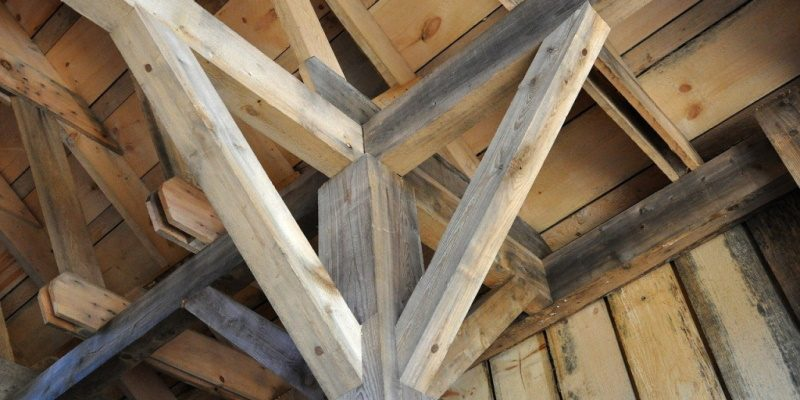In certain situations, we need to specify the correct angled cuts for the ends of our bracing material to fit perfectly. We can determine these angles using some basic woodworking tools. We can also use some trigonometry equations to calculate the cutting angles and any other related measurements.

🙋 If you want to learn more about wood beams and how to choose the correct wood species, grade, and size so you can avoid the need for knee bracings, you can check out our wood beam span calculator.

## How to calculate angle cuts?

When installing knee bracings, you may encounter many different situations that will lead to different starting points for your calculations. But don't worry, we will only be needing a few basic trigonometry concepts that work regardless of your starting point.

We also need to know the thickness of your plank or bracing material. This thickness should be consistent throughout the entire length of the plank. Once that is all set, we can now proceed to some of the different situations we can encounter.

If you have a limited space to place your knee bracing, determine this space's measurements first and use it to calculate the length of bracing you will need. Using the hypotenuse formula, which is based on the Pythagorean theorem, we can find the outer length of our knee bracing as follows:

$C = \sqrt{A^2 + B^2},$

where,

• $C$ – The outer length of our knee bracing;
• $A$ – The vertical measurement from where the post and beam intersect to the tip of the knee bracing attached to the post; and
• $B$ – The horizontal measurement from where the post and beam intersect to the tip of the knee bracing attached to the beam.

This formula can be used to solve the inner length of the knee bracing, too, if you know the inner measurements of the space you have. We can also utilize the Pythagorean theorem to solve for either length $A$ or length $B$ if we set a specific outer length for our knee bracing. You can learn more about this in the section of our Pythagorean theorem calculator.

After finding the lengths $A$, $B$, and $C$, or just any two of these lengths, we can also determine the angle cuts $\alpha$ and $\beta$ using the inverse of the sine, cosine, or the tangent formulas.

To better understand how to use these formulas, let us jump straight into an example.

## Finding the cutting angles sample calculation

Let's say we need to install additional knee bracing supports 40 centimeters from the beam of an open shed framing, as shown below: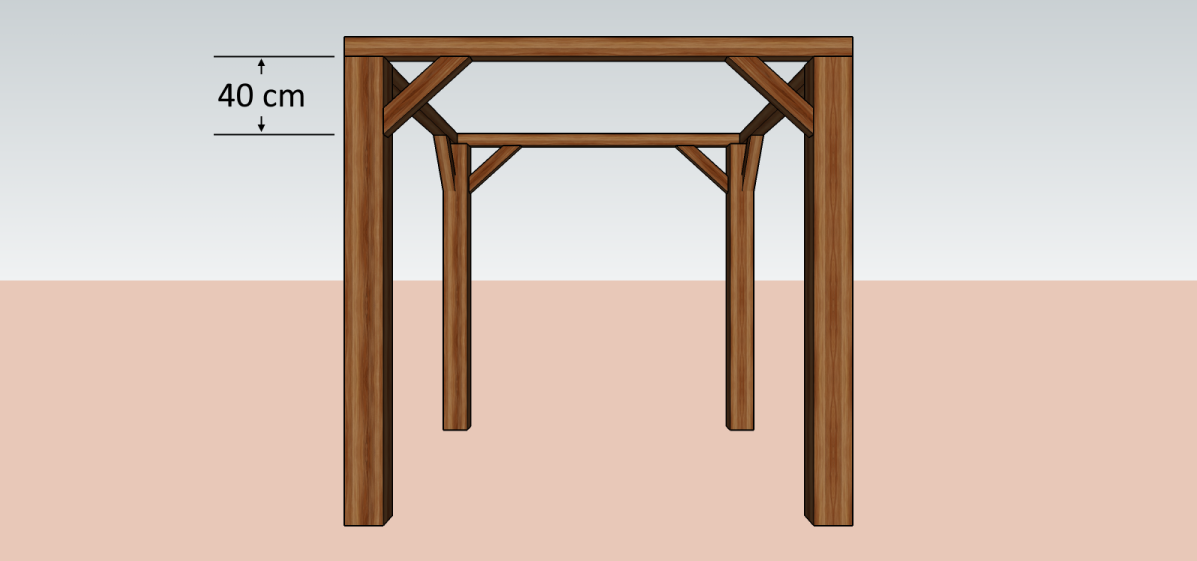If we need to use as much of 60-centimeter long and 10-cm wide planks as possible for our knee bracings, we can find the angle cuts by considering the right triangle formed by the open shade framing and our other known measurements, as shown below: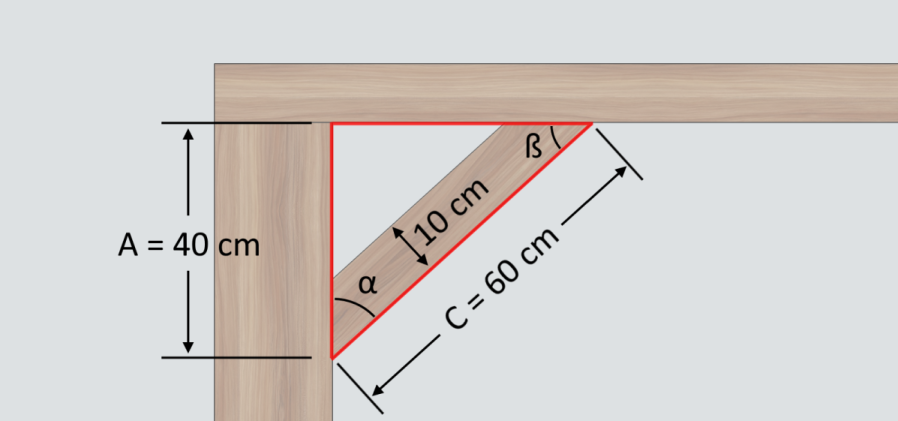Using the given measurement values, we can calculate angle α using the trigonometric function of arccosine as follows:

\small \begin{align*} \text{angle}\ \alpha &= \arccos\left(\frac{A}{C}\right)\\\\ &= \arccos\left(\frac{40\ \text{cm}}{60\ \text{cm}}\right)\\\\ &= 48.1896851\degree\\ &≈ 48.19\degree\text{✔} \end{align*}

Due to the nature of the right triangles, we can easily calculate the value of $\text{angle}\ \beta$ by finding the complementary angle of $\text{angle}\ \alpha$:

\small \begin{align*} \text{angle}\ \beta &= 90\degree - \text{angle}\ \alpha\\ &= 90\degree - 48.1896851\degree\\ &= 41.8103149\degree\\ &≈ 41.81\degree\text{✔} \end{align*}

If you do not have a protractor in your workshop, here is another method on how to cut wood at an angle. You can use the cutting angles $\alpha$ and $\beta$ to determine other knee bracing measurements and mark them accordingly before cutting. By considering the illustration below showing the similar right triangles within the knee bracing, you can then mark where to cut on your plank: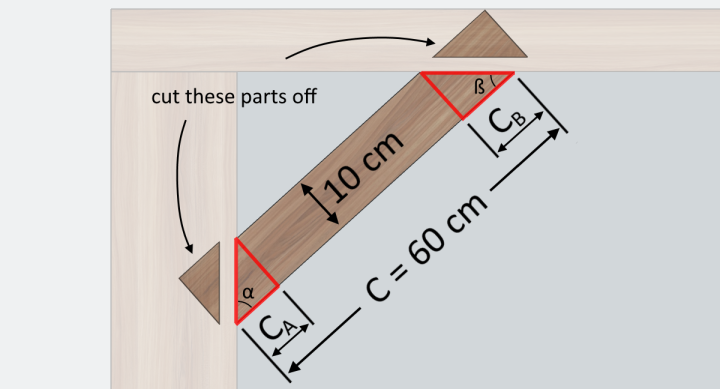We can calculate the values of small right triangle sides, $C_A$ and $C_B$, using the trigonometric function tangent and the thickness of the plank, as shown below:

\small \begin{align*} C_A &= \text{plank thickness} \div \tan(\alpha)\\ &= 10\ \text{cm} \div \tan(48.1896851\degree)\\ &= 8.94427191\ \text{cm}\\ &≈ 8.944\ \text{cm ✔} \end{align*}
\small\begin{align*} C_B &= \text{plank thickness} \div \tan(\beta)\\ &= 10\ \text{cm} \div \tan(41.8103149\degree)\\ &= 11.18033989\ \text{cm}\\ &≈ 11.18\ \text{cm ✔} \end{align*}

Now that we've determined all the details of our knee bracings, we can now cut the planks and install them afterward.

🙋 Wait! If you plan to butt your knee bracings' ends on the surface of the post and beam, you can go ahead and cut those ends. However, if you plan to use mortise and tenon for your joints, make sure only to mark off these ends and cut only portions of them to leave your desired mortise.

## Using our angle cut calculator

From our example above, you can instantly determine the various dimensions and measurements of the knee bracing by entering the given values of plank thickness, length $A$, and length $C$ in our angle cut calculator.

To properly use our calculator, make sure you enter the plank thickness (necessary) and any other two known measurements to find the unknown measurements. For best results, refresh the calculator when trying a new set of values.

Apart from knee bracings in wood and knee bracing in steel structures, you can also use our angle cut calculator for roof trusses and when installing supports for the formworks of retaining walls. You can learn more about roof trusses and retaining walls in our roof truss calculator and our retaining wall calculator

If you want to learn more about cutting boards and planks at an angle, perhaps you would like to check out our miter angle calculator. In our miter angle calculator (which is another woodworking angle finder), you will learn about what miter cut angle is, how it is different from miter saw angle, and so much more.

Kenneth Alambra
Bracing detail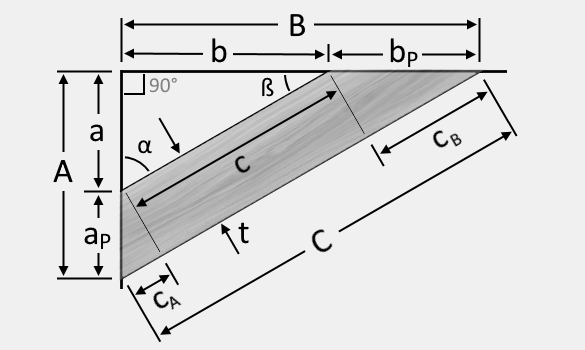Plank thickness, t
in
Bracing dimensions (input any three values)
Outer part distance, A
in
Inner part, distance a
in
Knee side, aₚ
in
Outer distance, B
in
Inner distance, b
in
Knee side, bₚ
in
Hit the reload button (the curved arrow below) to input new measurements.
Bracing angle
Angle α
Angle ß
Cut your plank in these dimensions
Outer length of plank, C
in
Inner length of the plank, c
in
Length Cᴀ
in
Length Cʙ
in
People also viewed…

### Car crash force

With this car crash calculator, you can find out how dangerous car crashes are.

### Christmas tree

Welcome to the Christmas tree calculator, where you will find out how to decorate your Christmas tree in the best way. Take a look at the perfect Christmas tree formula prepared by math professors and improved by physicists. Plan in advance how many lights and decorations you'll need!

### Concrete block fill

This concrete block fill calculator will help you determine how much concrete or mortar is needed to fill the void spaces inside a hollow concrete block wall of any size.

### French drain

Find out how much gravel, how many pipes, and optional filter fabric you need for any French drain project using this French drain calculator.